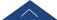﻿ 20220414 陈化 Eigenvalue Problems for Degenerate Elliptic Operators on Non-equiregular Sub-Riemannian Manifolds-湖南大学数学学院

# 20220414 陈化 Eigenvalue Problems for Degenerate Elliptic Operators on Non-equiregular Sub-Riemannian Manifolds

Sub-Riemannian Manifolds

self-adjoint Hormander operators on non-equiregular sub-Riemannian manifolds.

Using the Rayleigh-Ritz formula and the sub-elliptic heat kernel estimates, we

establish the upper bounds of eigenvalues which depend on the volume of subunit

ball and the measure of the manifold. Under a certain condition, we obtain the explicit

upper bounds of eigenvalues which have the polynomial growth in k with the optimal

order related to the non-isotropic dimension of the manifold.